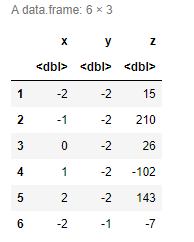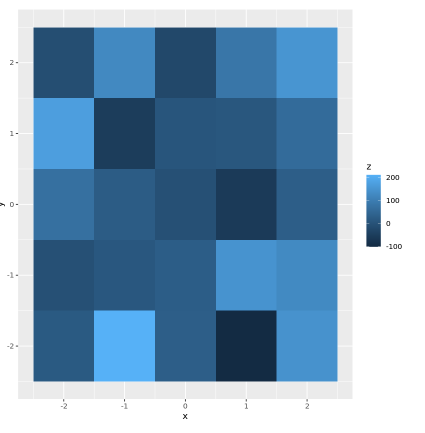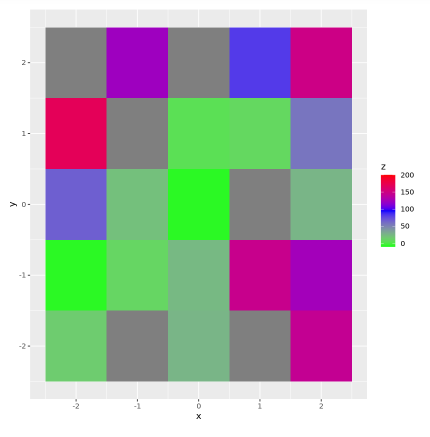# Scale ggplot2 Color Gradient to Range Outside of Data in R

• Last Updated : 03 May, 2022

In this article, we are going to see how to Scale ggplot2 Color Gradient to Range Outside of Data in R Programming Language.

### Creating dataframe:

Under this, we are simply creating a data frame of 6 rows and 3 columns.

## R

 `df <- ``data.frame``(x = ``seq``(- 2, 2, 1),``                   ``y = ``rep``(``seq``(- 2, 2, 1), each = 5),``                   ``z = ``round``(``rnorm``(25, 50, 70)))``head``(df)`

Output:## Create a graphic of the data

We are using the ggplot function from the ggploe2 library to plot the graph of the given dataframe. Here, we are just visualizing the data frame accordingly in the R programming language.

## R

 `library``(``"ggplot2"``)             ``new_df <- ``ggplot``(df,          ``              ``aes``(x, y, fill = z)) +``  ``geom_tile``()``new_df`

Output:## Specify Colors, Limits & Breaks Using scale_fill_gradient() Function:

In this method, the starting and the ending value of the colors to define a range is given as an argument.

Parameter:

• low: starting value
• high: ending value
• guide: type of legend

Example:

In this example, we are using the scale_fill_gradient() function to scale ggplot2 Color Gradient to Range Outside of Data bypassing the required parameter to the function in R programming language,

## R

 `new_df +                         ``  ``scale_fill_gradient``(colors = ``c``(``"Green"``, ``"Blue"``, ``"Red"``),``                       ``limits = ``c``(- 10, 200))`My Personal Notes arrow_drop_up